### 7 Examples

This section contains various examples of Lagrangian Coherent Structures in time-dependent dynamical systems:

7.4 Lobe dynamics revealed in fluids experiment (coming soon!)

To supplement this section, we will later cover in Sec.8 how FTLE fields computed, so the reader will know how the results shown below are produced. If so desired, Sec.8 on the Computation of FTLE's can be read before this section. Additionally, there is publicly available software, MANGEN, which provides a nice array of tools for analyzing dynamical systems defined by discrete data, including the capability to compute FTLE fields. We will show how to download this software, and walk through the computations shown in Sec.7.3 for flow over an airfoil.

#### 7.1 Time-dependent double gyre

Let's start with an example similar to the double gyre presented in Sec. 4 by Eq. (13). The flow is described by the stream-function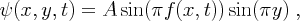(46)

where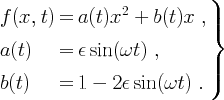(47)

over the domain [0, 2] x [0, 1]. The velocity field is given by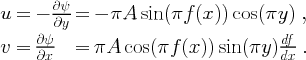(48)

For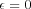the flow is time-independent and has the same form as Eq. (13) of Sec. 4. For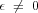the gyres conversely expand and contract periodically in the x-direction such that the rectangle enclosing the gyres remains invariant. In Eq. (46), A determines the magnitude of the velocity vectors,is the frequency of oscillation, andis approximately how far the line separating the gyres moves to the left or right, that is, the amplitude of the motion of the separation pointon the x-axis about the point (1,0) is given by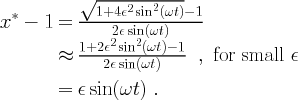Movie 11 shows the velocity field of the periodic double-gyre for A = 0.1,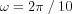, and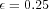. Notice that the period of motion is equal to 10 for this case.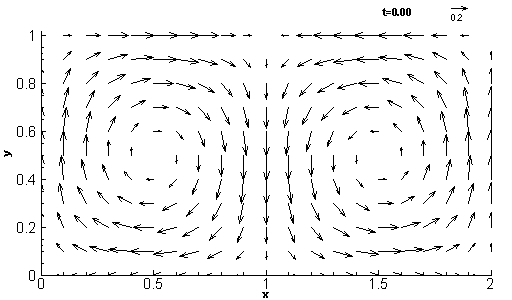Mov. 11: The double-gyre velocity field for A = 0 .1,, and.

Recall that for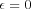the stable manifold of the fixed point (1, 0) and the unstable manifold of the fixed point (1, 1) form a heteroclinic connection. Such heteroclinic connections are unstable and are easily broken by the addition of small perturbations in time. This leads to classic entanglement of the unstable and stable manifolds; for references on this behavior see for example Ch.6 of  Ottino (1989) or Ch.4 of Guckenheimer and Holmes (1983). This classic entanglement geometry is nicely shown by the two movies below. However, the perturbation strength, measured bywas chosen to be quite large (= 0.25) to demonstrate that the LCS theory is not restricted to near-integrable systems. In fact, later examples will show the theory applied to turbulent flows.

Movie 12(a) shows the FTLE field computed forward in time with an integration time length of T = 15. Movie 12(b) shows the FTLE field computed backward in time with an integration time length of T = -15. Notice that the flow here is periodic with period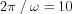and therefore the FTLE field is periodic with the same period of motion. Thus, in Movie 12(a) the FTLE field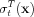is shown for t = 0 to t = 10 and Movie 12(b) shows the field for t = 10 to t = 0. In Movie 12(a) the ridge in the FTLE field, i.e. the LCS, represents the "stable manifold" and in Movie 12(b) the LCS represents the "unstable manifold". Notice that the attachment points of these manifolds do not correspond to the instantaneous stagnation (fixed) points of the Eulerian velocity field. For example, at time t = 0 or time t = 10, the instantaneous fixed points are located at (1,0) and (1,1), however neither LCS attaches to the boundaries at these points. This helps demonstrate why trying to "grow" manifolds from instantaneous fixed points is often troublesome for time-dependent systems.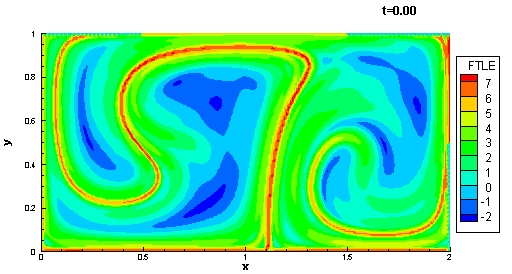(a) T = 15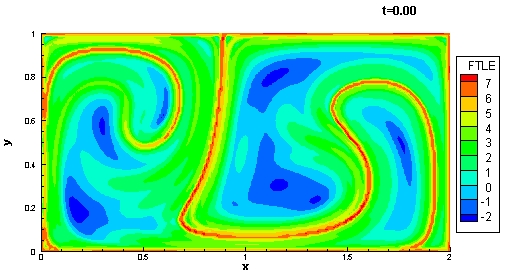(b) T = -15
 Mov. 12: FTLE fields for time-varying double gyre with A = 0.25,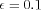, andTo demonstrate the fact that the LCS shown in Movie 12 are invariant manifolds (or at least quasi-invariant), let us place a material point which moves according to the flow on top of the LCS shown in Movie 12(a). Movie 13 shows the time evolution of the superposition of this material point, denoted by an X, along with the LCS. The initial location of the material point was "eyeballed" to be initially placed on the LCS. From the movie, one can see that the point stays on the LCS when evolved over time. In Movie 13 the LCS is highlighted by plotting the FTLE field using a bi-color contour map in which high values of FTLE's are colored red, and low values colored white. This allows the LCS to be highlighted, and also permits us to better superimpose the evolution of other data. We use similarly skewed contour maps in later examples when showing FTLE fields to help highlight the LCS and keep the rest of the contour plot transparent.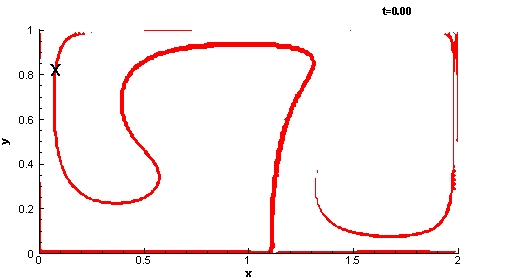Mov. 13: Evolution of the LCS and a Lagrangian tracer over time time. The tracer is denoted by the X (Computed with A = 0.1,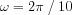,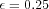).

In Movie 14 we now superimpose two parcels of fluid particles, which are initially located on either side of the LCS, with the evolution of the LCS. This movie demonstrates how the LCS acts as a time-dependent separatrix. The parcel which begins to the left of the LCS gets stretched into the left gyre and the parcel that begins on the right side of the LCS gets stretched into the right gyre.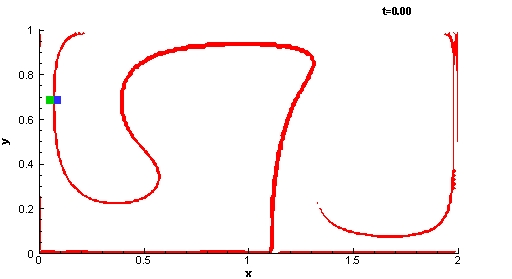Mov. 14: Time evolution of the LCS along with two parcels of fluid particles initially located on either side of the LCS. (Computed with A = 0.1,,).

Although the LCS appears to be an invariant manifold from the above movies, we know from Thm.6.1 that there may be a slight flux across this structure. Figure 15 shows a highly refined computation of the LCS and the location of the Lagrangian tracer shown in Movie 13. The grid spacing that was used for the computation of FTLE was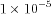. Computations reveal that the tracer moves at an average rate of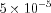normal to the LCS over the interval considered. This rate is about 0.05% of the magnitude of the velocity field in that region. This amount is exceedingly small and well below tolerable numerical error for most practical analyses.

To verify Thm. 6.1, the terms in the right-hand-side of Eq. (22) were computed from a first-order approximation. The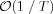term dominates for this example with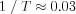(the other terms of Eq.(22) are much smaller than 0.03 when evaluated). The results confirm Eq. (22) since the "directly computed" flux of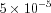is well below.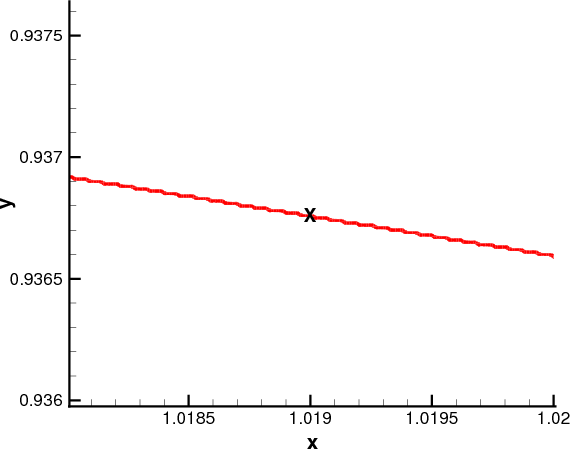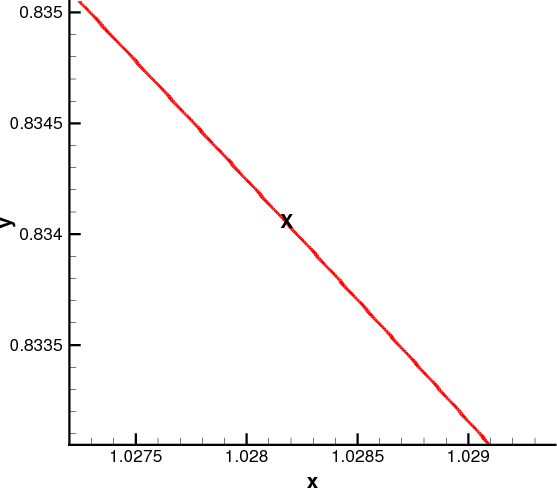(a) t = 0                                                               (b) t = 1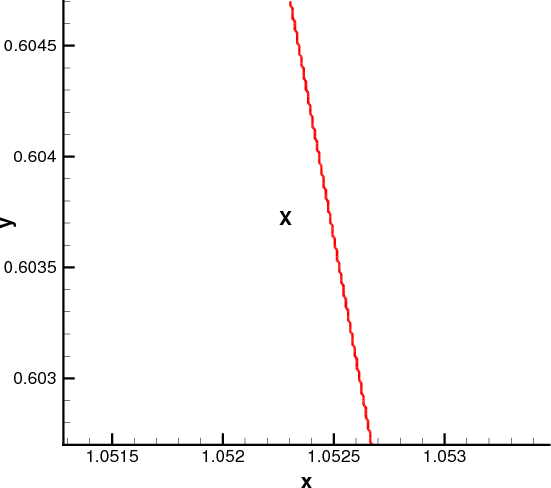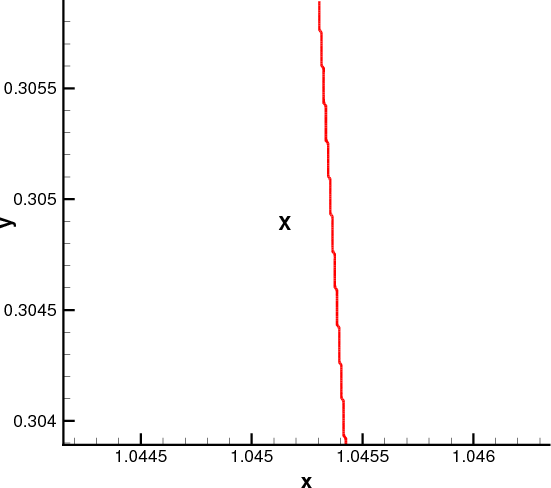(c) t = 2                                                              (d) t = 3
 Fig. 15: Highly refined plots of the locations of the LCS and the Lagrangian tracer at 4 different times. Note the very small grid spacing used in these plots. (Computed with A = 0.1,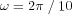,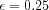, T = 30, and a grid spacing for FTLE computations of 10-5.)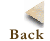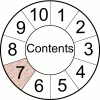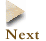Comments, Questions or Concerns can be sent to shawn@cds.caltech.edu